Courses

# Data Interpretation MCQ -5

## 20 Questions MCQ Test General Aptitude for GATE 2020 | Data Interpretation MCQ -5

Description
This mock test of Data Interpretation MCQ -5 for Quant helps you for every Quant entrance exam. This contains 20 Multiple Choice Questions for Quant Data Interpretation MCQ -5 (mcq) to study with solutions a complete question bank. The solved questions answers in this Data Interpretation MCQ -5 quiz give you a good mix of easy questions and tough questions. Quant students definitely take this Data Interpretation MCQ -5 exercise for a better result in the exam. You can find other Data Interpretation MCQ -5 extra questions, long questions & short questions for Quant on EduRev as well by searching above.
QUESTION: 1

### (Q.no: 1-5). Refer to the following Line Graph and answer the given questions.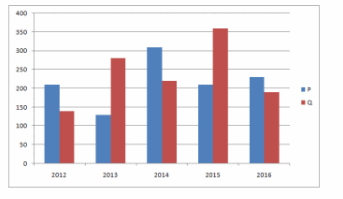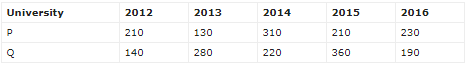In 2013, out of the total number of applications received for Universities P and Q together, only 30% were accepted. What was the total number of applications accepted for Universities P and Q together in 2013?

Solution:

Total number of applications accepted by P and Q together in 2013 = (130 + 280)*30/100
=123

QUESTION: 2

### (Q.no: 1-5). Refer to the following Line Graph and answer the given questions.In 2012, 30% of applications received for University P and 20% of applications received for University Q were from international students. What was the total number of International applicants for University P and Q together in 2012?

Solution:

Total Number of International applicants for University P and Q together = 210*30/100 + 140 * 20/100
= 63 + 28 = 91

QUESTION: 3

### (Q.no: 1-5). Refer to the following Line Graph and answer the given questions.If the respective ratio of total number of applications received for University P and Q together in 2016 and 2017 is 3:4. What was the total number of applications received for University P and Q together in 2017?

Solution:

Total number of applications received for Universities P and Q together in 2016 = 230 + 190 = 420
Total number of applications received for Universities P and Q together in 2017 = 420 * 4/3 = 560

QUESTION: 4

(Q.no: 1-5). Refer to the following Line Graph and answer the given questions.What is the average number of applications received for University P in 2013, 2015 and 2016?

Solution:

Average number of applications received for University A in 2013, 2015 and 2016 = (130 + 210 + 230)/3 = 190

QUESTION: 5

(Q.no: 1-5). Refer to the following Line Graph and answer the given questions.Number of applications received for University Q increased by what percent from 2012 to 2014?

Solution:

% = (220-140)/140 * 100 = 400/7 %

QUESTION: 6

(Q.no: 6-10). Refer to the following Line Graph and answer the given questions.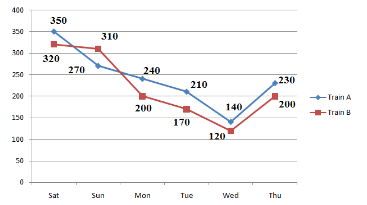The number of people who traveled by Train B on Friday is 30% more than the people who traveled by the same train on Thursday. What is the respective ratio between the number of people who traveled on Friday and those who traveled on Saturday by the same train?

Solution:

30% of 200 = 60
People traveled by Train B on Friday = 260
260 : 320 = 13 : 16

QUESTION: 7

(Q.no: 6-10). Refer to the following Line Graph and answer the given questions.What is the difference between the total number of people who traveled by Train B on Saturday and Sunday together and the total number of people who traveled by Train A on Saturday and Sunday together?

Solution:

Train B on Saturday and Sunday together = 320 + 310 = 630
Train A on Saturday and Sunday together = 350 + 270 = 620
Difference = 630 – 620 = 10

QUESTION: 8

(Q.no: 6-10). Refer to the following Line Graph and answer the given questions.What is the average number of people traveling by Train B on Monday, Tuesday, Wednesday and Thursday?

Solution:

200 + 170 + 120 + 200
= 172.5

QUESTION: 9

(Q.no: 6-10). Refer to the following Line Graph and answer the given questions.The number of people who traveled by Train A decreased by what percent from Saturday to Wednesday?

Solution:

[(350 – 140)/350]*100
= [210/350]*100 = 60%

QUESTION: 10

(Q.no: 6-10). Refer to the following Line Graph and answer the given questions.The total number of people who traveled by both the given trains together on Monday is approximately what percent more than the total number of people who traveled by both the given trains together on Wednesday?

Solution:

(440 – 260)/260*100
= 180/260 * 100
= 69%

QUESTION: 11

(Q.no: 15-20). Refer to the table and answer the given questions.
Total Population in Five Cities = 15,00,000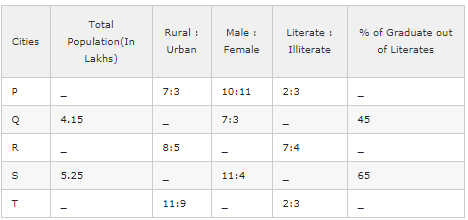The total population of City P and R is 7,85,000. The difference between the total population of City P and R is 2.65 lakhs. If the City P has more population than City R then the urban population of City P and R together is approximately what percent of total population of these two Cities?

Solution:

P + R = 7,85,000
(R has more population than P) R – P = 265000
P = 5,25,000 ; R = 2,60,000
Urban population of City P and R = 3/10 * 5,25,000 + 5/13 * 2,60,000 = 257500
% = (257500 / 785000)*100 = 32%

QUESTION: 12

(Q.no: 15-20). Refer to the table and answer the given questions.
Total Population in Five Cities = 15,00,000The difference between the number of male and female in the City P is 10000. Approximately, by what percent of urban population of City P less than its Rural population?

Solution:

X = Total population in City A
1/21 * x = 10000 => x = 2,10,000
Rural Population = 1,47,000
Urban Population = 63,000
% = (Difference / Rural Population)*100 = (84,000 / 147000)*100 = 57%

QUESTION: 13

(Q.no: 15-20). Refer to the table and answer the given questions.
Total Population in Five Cities = 15,00,000One – fourth of total population of City R is 2,20,000.  If the total number of Literate graduates of City Q and S are 22,500 and 52,000. What % of the total population of City Q, R and S together is literate?

Solution:

1/4 * R = 2,20,000 => R = 8,80,000
Literate population of City R = 7/11 * 8,80,000 = 5,60,000
Total number of Literate graduates of City Q = 22500 = 45%
x = Literate population of City Q
45% of x = 22500 => x = 50000
Total number of Literate graduates of City S = 52000 = 65%
y = Literate population of City S
65% of y = 52000 => x = 80000
% = (690000/1820000) * 100 = 37.9%

QUESTION: 14

(Q.no: 15-20). Refer to the table and answer the given questions.
Total Population in Five Cities = 15,00,000The difference between the total population of City R and T is 30,000. The ratio of total population of City R and T is 44:41. Total % of Literate graduates of City R and T is 90%. If the % Literate graduates of City T is equalled to two times of Literate graduates of City R then what is the difference between the number of graduates from City R and City T?

Solution:

3/85 * x = 30000 => x = 850000
44/85*850000 = 440000 = City R Population
7/11*440000 = 280000
Number of graduates from City R = 30% of 280000 = 84000
41/85*85000 = 410000 = City T Population
2/5*410000 = 164000
Number of graduates from City T = 60% of 1,64,000 = 98400
Difference = 14400

QUESTION: 15

(Q.no: 15-20). Refer to the table and answer the given questions.
Total Population in Five Cities = 15,00,000What is the sum of female population in City Q and male population in City S together ?

Solution:

Sum = 3/10 * 415000 + 11/15 *525000 = 124500 + 385000 = 509500

QUESTION: 16

(Q.no: 16 -20). Refer to the table and answer the given questions.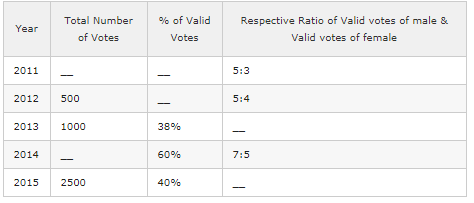In 2013, if the number of valid votes of female was 100, what was the respective ratio of number of valid votes of male and number of valid votes of female same year?

Solution:

38% of 1000 = 380
Valid votes of Female = 100
Valid votes of Male = 380 – 100 = 280
=> 280:100
=> 14:5

QUESTION: 17

(Q.no: 16 -20). Refer to the table and answer the given questions.The total number of votes increased by 50% from 2012 to 2016. If 16% of the votes valid in 2016, what was the number of valid votes in 2016?

Solution:

50% of 500 = 250
= 500 + 250 = 750
16% of 750 = 120

QUESTION: 18

(Q.no: 16 -20). Refer to the table and answer the given questions.If the average number of valid votes in 2012 and 2015 was 635, approximately, what percent of total votes valid in 2012?

Solution:

Number of valid votes in 2012 and 2015 = 1270
2015: 40% of 2500 = 1000
1270 – 1000 = 270
(270/500)*100 = 54%

QUESTION: 19

(Q.no: 16 -20). Refer to the table and answer the given questions.In 2014, if the difference between number of valid votes of male and number of valid votes of female was 150, what was the total number of votes in 2014?

Solution:

(1/6)*x = 15
x = 900
60% of y = 900
y = 1500

QUESTION: 20

(Q.no: 16 -20). Refer to the table and answer the given questions.In 2011, the respective ratio of total number of votes to valid votes was 5:4. Number of valid votes of female in 2011 constitutes what percent of the total number of votes in the same year?

Solution:

[4x * (3/8) / 5] * 100 = 30%